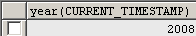Tutorials

# Mysql Date Get Year

Mysql Date Get Year is the way of retrieving the current year.

Mysql Date Get Year is the way of retrieving the current year.

# Mysql Date Get Year

Mysql Date Get Year is the way of retrieving the current year.

Understand with Example

The Tutorial illustrate an example from 'Mysql Date Get Year'. To understand and grasp this example, we use the following syntax:

year(CURRENT_TIMESTAMP ): This Query  returns the current year. This is synonyms for NOW().

Query:-

 Select year(CURRENT_TIMESTAMP);

Output:-Another way to get the current year is:-

The given below Query return you the current year as field name year(now( )).

Query:-

 SELECT year(now());

now():-This is the inbuilt function in the MySql which returns the current Date and Time of the System.

Output:-